StatLect

# Support of a random variable

The support of a random variable is the set of values that the random variable can take.## Support of a discrete variable

For discrete random variables, it is the set of all the realizations that have a strictly positive probability of being observed.

Example If a discrete random variable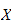has probability mass function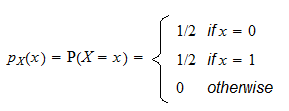its support, denoted by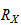, is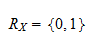## Support of a continuous variable

For continuous random variables, it is the set of all numbers whose probability density is strictly positive.

Example If a continuous random variablehas probability density function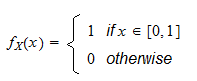then its support is## Support of random vectors and random matrices

The same definition applies to random vectors. Ifis a random vector, its supportis the set of values that it can take. The concept extends in the obvious manner also to random matrices.

## Synonyms

The support is sometimes also called range.

## More details

The lecture entitled Random variables explains the concept of support in more detail.

Previous entry: Statistical model

Next entry: Test statistic